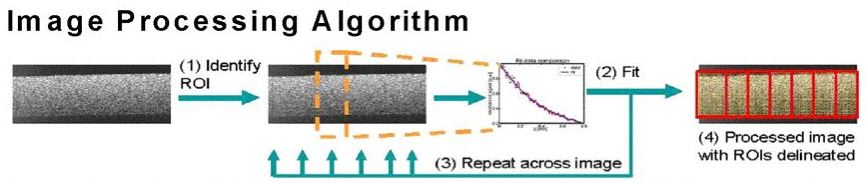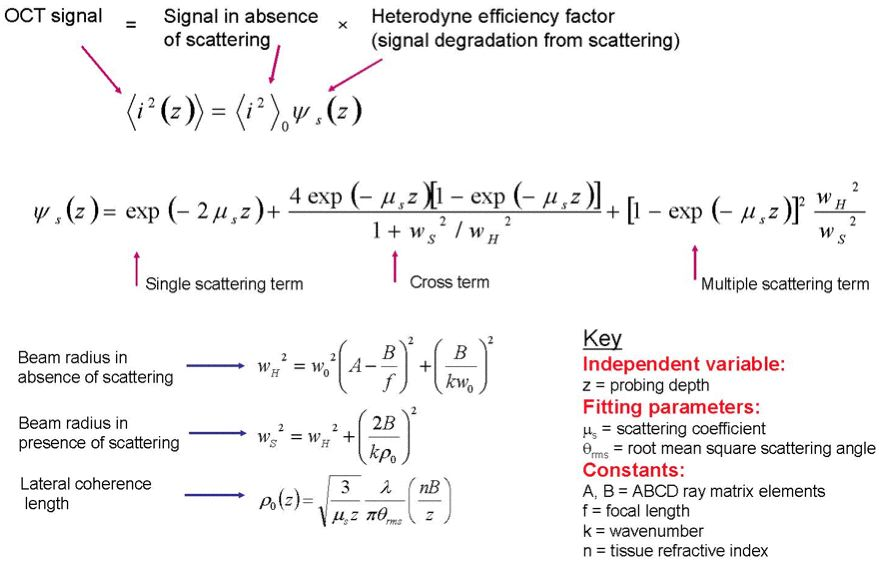# Methods: OCT Image Processing Algorithm

A schematic of our extraction algorithm is shown in Fig. 2 below. Briefly summarizing the algorithm, first, an OCT image is laterally divided into regions of interest (ROIs). In each ROI, we fit the laterally averaged OCT signal to a theoretical model and iterate the scattering coefficient μs and root mean square scattering angle θrms which characterize optical scattering in that ROI. The effective anisotropy parameter geff is then calculated using the relationship geff = cos θrms. The algorithm returns the OCT image with ROIs delineated and labelled with their respective optical properties.Fig. 2: Our extraction algorithm. (1) The user delineates an ROI in an OCT image. (2) The signal is averaged, fit, and local μs and θrms are iterated. (3) The user delineates successive ROIs across the image. (4) Processed image and numerical output are returned.

Our extraction algorithm utilizes a general theoretical model that takes into account multiple scattering effects and considers sample arm geometries under realistic focusing conditions. In this experiment, a time domain OCT system operating at 1310 nm was used, with light focused on the proximal glass-collagen interface. The fitting equations in the algorithm are shown in Fig. 3. Details on our algorithm and theoretical model are found in Refs.  and , respectively.Fig. 3: Equations governing the extraction algorithm.

References:
1. D. Levitz, L. Thrane, M. H. Frosz, P. E. Andersen, C. B. Andersen, J. Valanciunaite, J. Swartling, S. Andersson-Engels, and P.R. Hansen, "Determination of optical scattering properties of highly-scattering media in optical coherence tomography images," Opt. Express 12, 249-259 (2004).

2. L. Thrane, H. T. Yura, and P. E. Andersen, "Analysis of optical coherence tomography systems based on the extended Huygens-Fresnel principle," J. Opt. Soc. Am. A 17, 484-490 (2000).